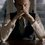# 1+2+3+4+5........infinity=-1/12, HOW?

This is something which made me amazed and astonished when I saw to it for the first time. Mathematics at its very best, truly! For proving it, we first need to prove that $${ S }_{ 1 }$$=1-1+1-1+1-1.......$$\infty$$=1/2

To prove this, first add ${ S }_{ 1 }$ to itself 2${ S }_{ 1 }$ = 1-1+1-1+1-1........$\infty$

                          +1-1+1-1+1........infinite


here comes the game changer, we wouldn't add directly to get our answer again to zero, rather, we would add to one no. forward. We would do calculations by neglecting the first no. and then the same. This wouldn't disturb our series as it is an infinite series

Now, we get 2${ S }_{ 1 }$=1

${ S }_{ 1 }$=1/2

Here, we would introduce another series ${ S }_{ 2 }$=1-2+3-4+5-6......$\infty$

Add it to itself 2${ S }_{ 2 }$=1-2+3-4+5.......$\infty$

                        +1-2+3-4........infinite      [same type of addition as did above]


$\therefore$ 2${ S }_{ 2 }$=1-1+1-1+1-1.....$\infty$

which would give

2${ S }_{ 2 }$=1/2(proved above ${ S }_{ 1 }$=1/2)

Hence,

${ S }_{ 2 }$=1/4

Now, ${ S }_{ 3 }$ = 1+2+3+4+5.........$\infty$

Subtract ${ S }_{ 2 }$ from ${ S }_{ 3 }$

${ S }_{ 3 }$ - ${ S }_{ 2 }$ = 1+2+3+4+5+6.........$\infty$ - [1-2+3-4+5-6.....$\infty$]

${ S }_{ 3 }$ - ${ S }_{ 2 }$ = 1+2+3+4+5+6.........$\infty$

                                              -1+2-3+4-5+6.............infinite


[typical addition, not as we did above]

We get,

${ S }_{ 3 }$ - ${ S }_{ 2 }$ = 0+4+0+8+0+12.........$\infty$

                                           = 4+8+12+16+20+24........infinite

= 4[1+2+3+4+5+6..........infinite


${ S }_{ 3 }$ - ${ S }_{ 2 }$ = 4${ S }_{ 3 }$ [we know that1+2+3..$\infty$=${ S }_{ 3 }$]

Put value of ${ S }_{ 2 }$=1/4 proved above in this equation

${ S }_{ 3 }$ - 1/4 = 4${ S }_{ 3 }$

• 1/4 = 3${ S }_{ 3 }$

$\therefore$ ${ S }_{ 3 }$ = -1/12

or

1+2+3+4+5.......$\infty$ = -1/12

Ha, that was something crazy and i got tired in writing that, as well. But mathematics never tire you, only this pc work does...... For the love of Mathematics!Note by Kartik Sharma
7 years, 3 months ago

This discussion board is a place to discuss our Daily Challenges and the math and science related to those challenges. Explanations are more than just a solution — they should explain the steps and thinking strategies that you used to obtain the solution. Comments should further the discussion of math and science.

When posting on Brilliant:

• Use the emojis to react to an explanation, whether you're congratulating a job well done , or just really confused .
• Ask specific questions about the challenge or the steps in somebody's explanation. Well-posed questions can add a lot to the discussion, but posting "I don't understand!" doesn't help anyone.
• Try to contribute something new to the discussion, whether it is an extension, generalization or other idea related to the challenge.

MarkdownAppears as
*italics* or _italics_ italics
**bold** or __bold__ bold
- bulleted- list
• bulleted
• list
1. numbered2. list
1. numbered
2. list
Note: you must add a full line of space before and after lists for them to show up correctly
paragraph 1paragraph 2

paragraph 1

paragraph 2

[example link](https://brilliant.org)example link
> This is a quote
This is a quote
    # I indented these lines
# 4 spaces, and now they show
# up as a code block.

print "hello world"
# I indented these lines
# 4 spaces, and now they show
# up as a code block.

print "hello world"
MathAppears as
Remember to wrap math in $$ ... $$ or $ ... $ to ensure proper formatting.
2 \times 3 $2 \times 3$
2^{34} $2^{34}$
a_{i-1} $a_{i-1}$
\frac{2}{3} $\frac{2}{3}$
\sqrt{2} $\sqrt{2}$
\sum_{i=1}^3 $\sum_{i=1}^3$
\sin \theta $\sin \theta$
\boxed{123} $\boxed{123}$

Sort by:

Well, nice work but convergence and divergence would have reduced the work and made it more beautiful.

- 3 years, 12 months ago